# How to Calculate and Solve for Total Formation Volume Factor | The Calculator Encyclopedia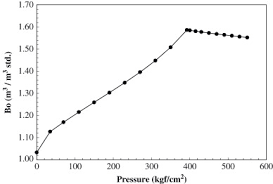The image above represents the total formation volume factor (FVF).

To compute for the total FVF, four essential parameters are needed and these parameters are oil FVF (Bo), gas FVF (Bg), initial gas solubility (Rsi) and current gas solubility (Rs).

The formula for calculating the total FVF:

BT = Bo + (Rsi – Rs)Bg

Where;

BT = Total FVF
Bo = Oil FVF
Bg = Gas FVF
Rsi = Initial Gas Solubility
Rs = Current Gas Solubility

Let’s solve an example;
Find the total FVF when the oil FVF is 14, gas FVF is 15, initial gas solubility is 26 and current gas solubility is 17.

This implies that;

Bo = Oil FVF = 14
Bg = Gas FVF = 15
Rsi = Initial Gas Solubility = 26
Rs = Current Gas Solubility = 17

BT = Bo + (Rsi – Rs)Bg
BT = 14 + (26 – 17)15
BT = 14 + (9)15
BT = 14 + 135
BT = 149

Therefore, the total FVF is 149.

Calculating the Oil FVF when the Total FVF, Gas FVF, Initial Gas Solubility and Current Gas Solubility is Given.

Bo = BT – (Rsi – Rs)Bg

Where;

Bo = Oil FVF
BT = Total FVF
Bg = Gas FVF
Rsi = Initial Gas Solubility
Rs = Current Gas Solubility

Let’s solve an example;
Find the oil FVF when the total FVF is 44, gas FVF is 17, initial gas solubility is 21 and current gas solubility is 19.

This implies that;

BT = Total FVF = 44
Bg = Gas FVF = 17
Rsi = Initial Gas Solubility = 21
Rs = Current Gas Solubility = 19

Bo = BT – (Rsi – Rs)Bg
Bo = 44 – (21 – 19)17
Bo = 44 – (2)17
Bo = 44 – 34
Bo = 10

Therefore, the oil FVF is 10.

Calculating the Gas FVF when the Total FVF, Oil FVF, Initial Gas Solubility and Current Gas Solubility is Given.

Bg = BT – Bo / Rsi – Rs

Where;

Bg = Gas FVF
Bo = Oil FVF
BT = Total FVF
Rsi = Initial Gas Solubility
Rs = Current Gas Solubility

Let’s solve an example;
Find the gas FVF when the total FVF is 48, oil FVF is 16, initial gas solubility is 20 and current gas solubility is 12.

This implies that;

Bo = Oil FVF = 16
BT = Total FVF = 48
Rsi = Initial Gas Solubility = 20
Rs = Current Gas Solubility = 12

Bg = BT – Bo / Rsi – Rs
Bg = 48 – 16 / 20 – 12
Bg = 32 / 8
Bg = 4

Therefore, the gas FVF is 4.

Calculating the Initial Gas Solubility when the Total FVF, Gas FVF, Oil FVF and Current Gas Solubility is Given.

Rsi = BT – Bo / Bg + Rs

Where;

Rsi = Initial Gas Solubility
BT = Total FVF
Bg = Gas FVF
Bo = Oil FVF
Rs = Current Gas Solubility

Let’s solve an example;
Find the initial gas solubility when the total FVF is 54, oil FVF is 14, gas FVF is 12 and current gas solubility is 24.

This implies that;

BT = Total FVF = 54
Bg = Gas FVF = 14
Bo = Oil FVF = 12
Rs = Current Gas Solubility = 24

Rsi = BT – Bo / Bg + Rs
Rsi = 54 – 12 / 14 + 24
Rsi = 42 / 14 + 24
Rsi = 3 + 24
Rsi = 27

Therefore, the initial gas solubility is 27.

Calculating the Current Gas Solubility when the Total FVF, Gas FVF, Oil FVF and  Initial Gas Solubility is Given.

Rs = RsiBT – Bo / Bg

Where;

Rs = Current Gas Solubility
Rsi = Initial Gas Solubility
BT = Total FVF
Bg = Gas FVF
Bo = Oil FVF

Let’s solve an example;
Find the current gas solubility when the total FVF is 14, oil FVF is 6, gas FVF is 10 and  initial gas solubility is 5.

This implies that;

Rsi = Initial Gas Solubility = 5
BT = Total FVF = 14
Bg = Gas FVF = 10
Bo = Oil FVF = 6

Rs = RsiBT – Bo / Bg
Rs = 5 – 14 – 6 / 10
Rs = 5 – 8 / 10
Rs = 5 – 0.8
Rs = 4.2

Therefore, the current gas solubility is 4.2

Nickzom Calculator – The Calculator Encyclopedia is capable of calculating the total FVF.

To get the answer and workings of the total FVF using the Nickzom Calculator – The Calculator Encyclopedia. First, you need to obtain the app.

You can get this app via any of these means:

To get access to the professional version via web, you need to register and subscribe for NGN 1,500 per annum to have utter access to all functionalities.
You can also try the demo version via https://www.nickzom.org/calculator

Apple (Paid) – https://itunes.apple.com/us/app/nickzom-calculator/id1331162702?mt=8
Once, you have obtained the calculator encyclopedia app, proceed to the Calculator Map, then click on Petroleum under EngineeringNow, Click on Reservoir Fluid Flow under Petroleum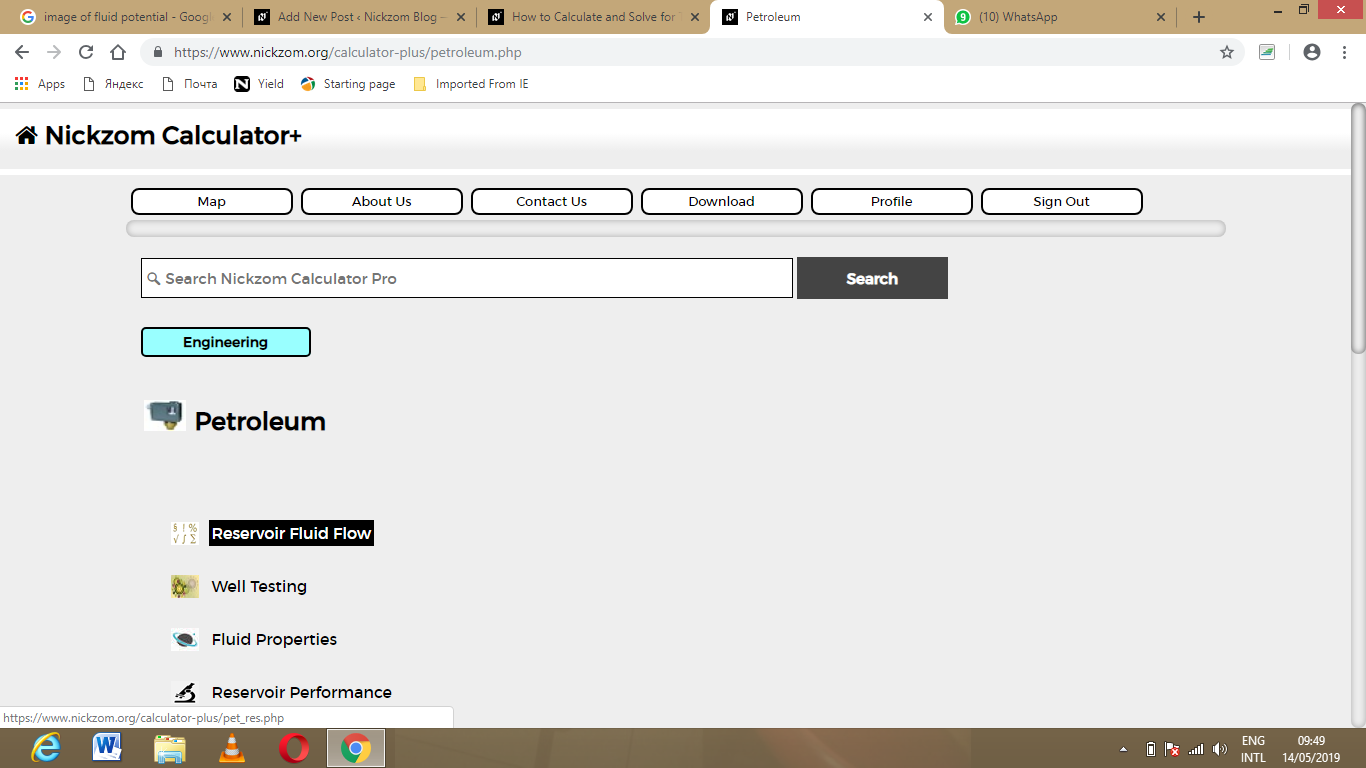Now, Click on Total FVF under Reservoir Fluid Flow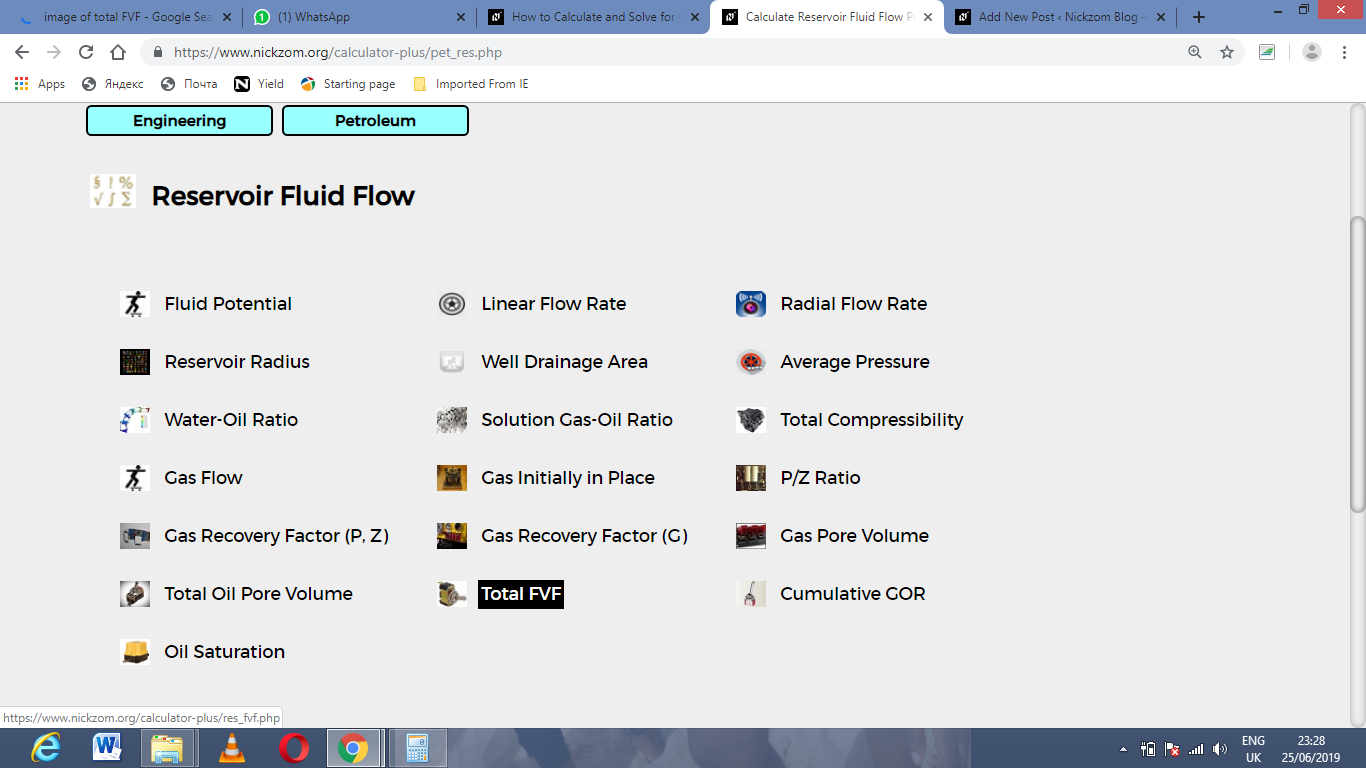The screenshot below displays the page or activity to enter your value, to get the answer for the total FVF according to the respective parameter which are the oil FVF (Bo), gas FVF (Bg), initial gas solubility (Rsi) and current gas solubility (Rs).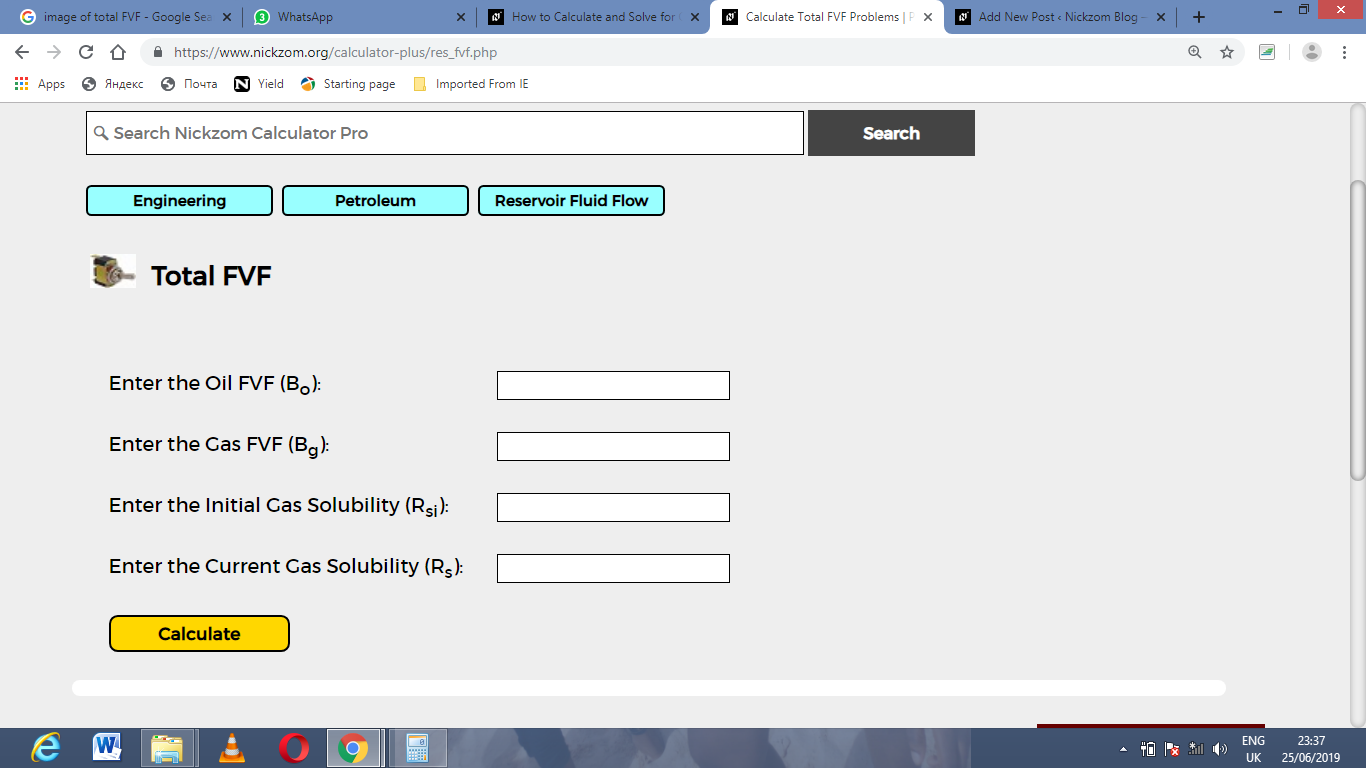Now, enter the value appropriately and accordingly for the parameter as required by the oil FVF (Bo) is 14, gas FVF (Bg) is 15, initial gas solubility (Rsi) is 26 and current gas solubility (Rs) is 17.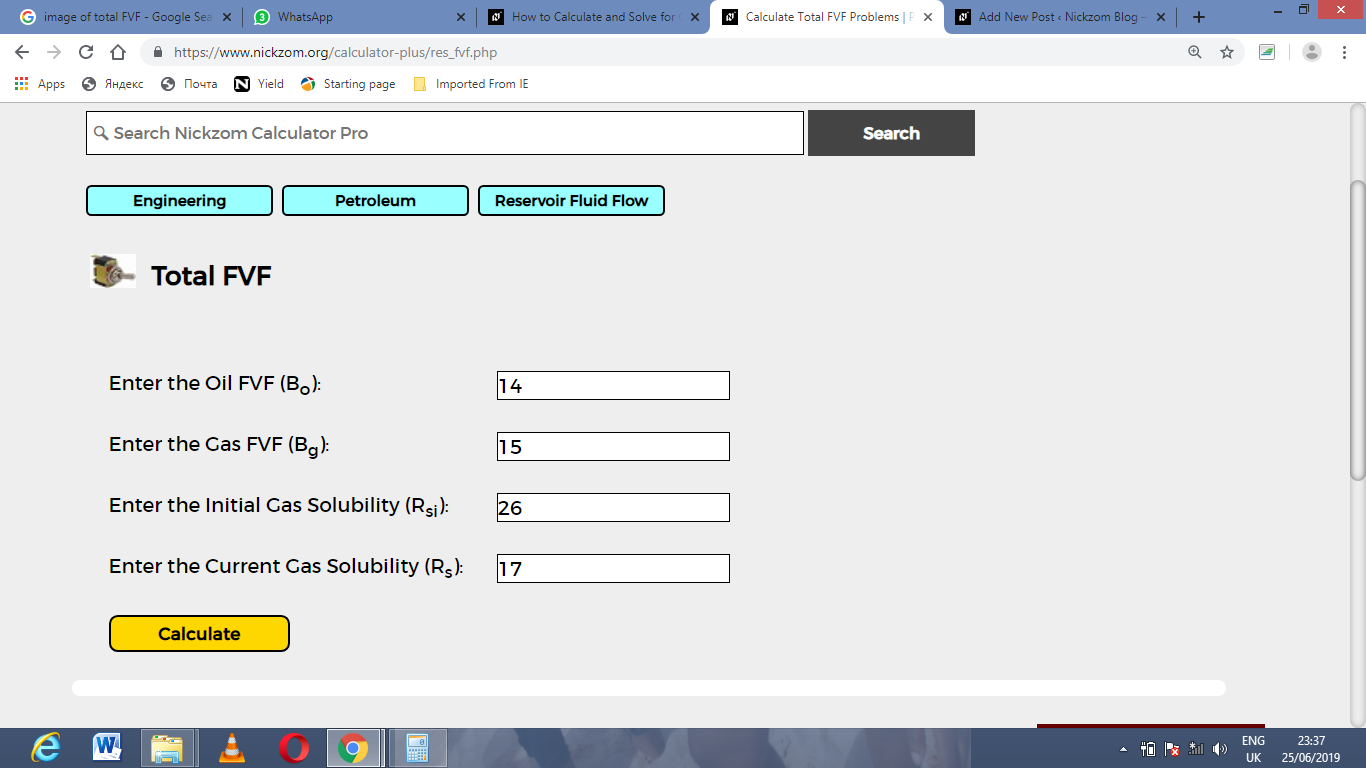Finally, Click on Calculate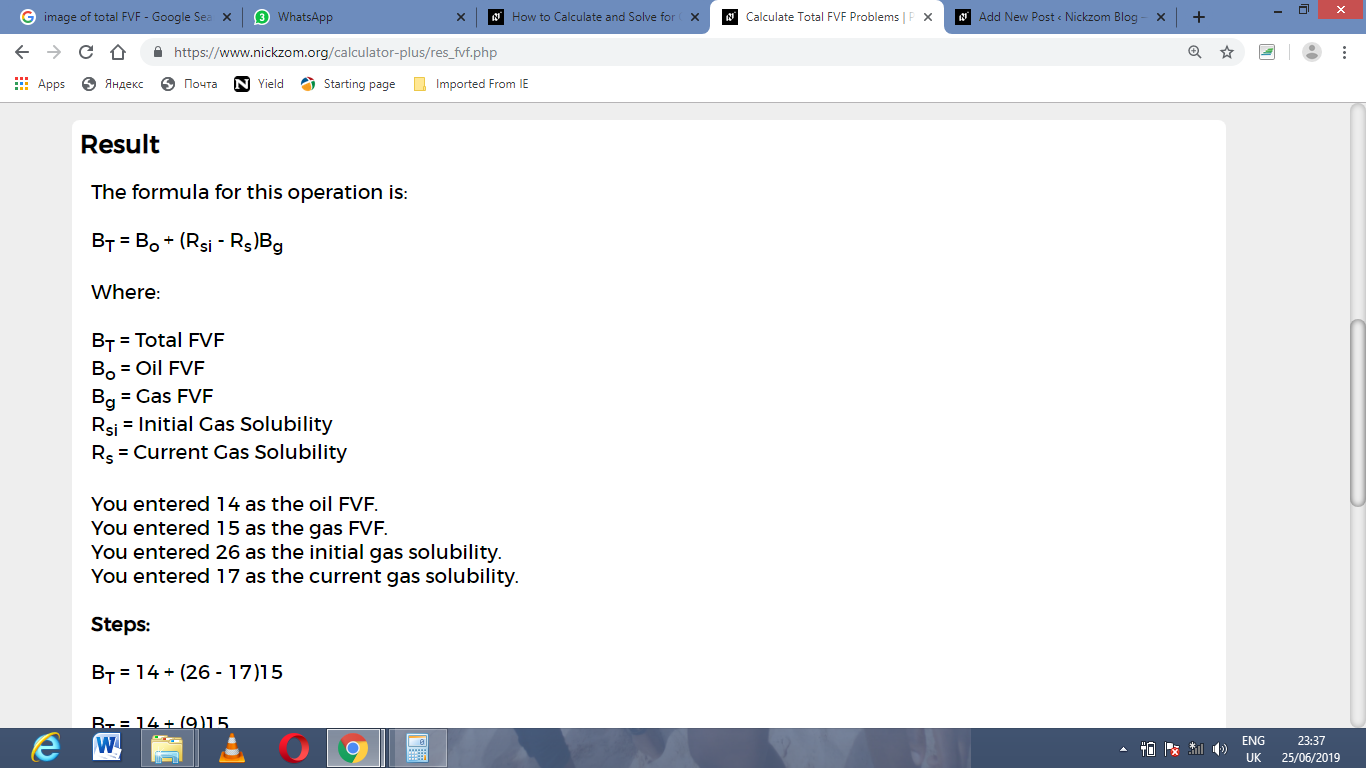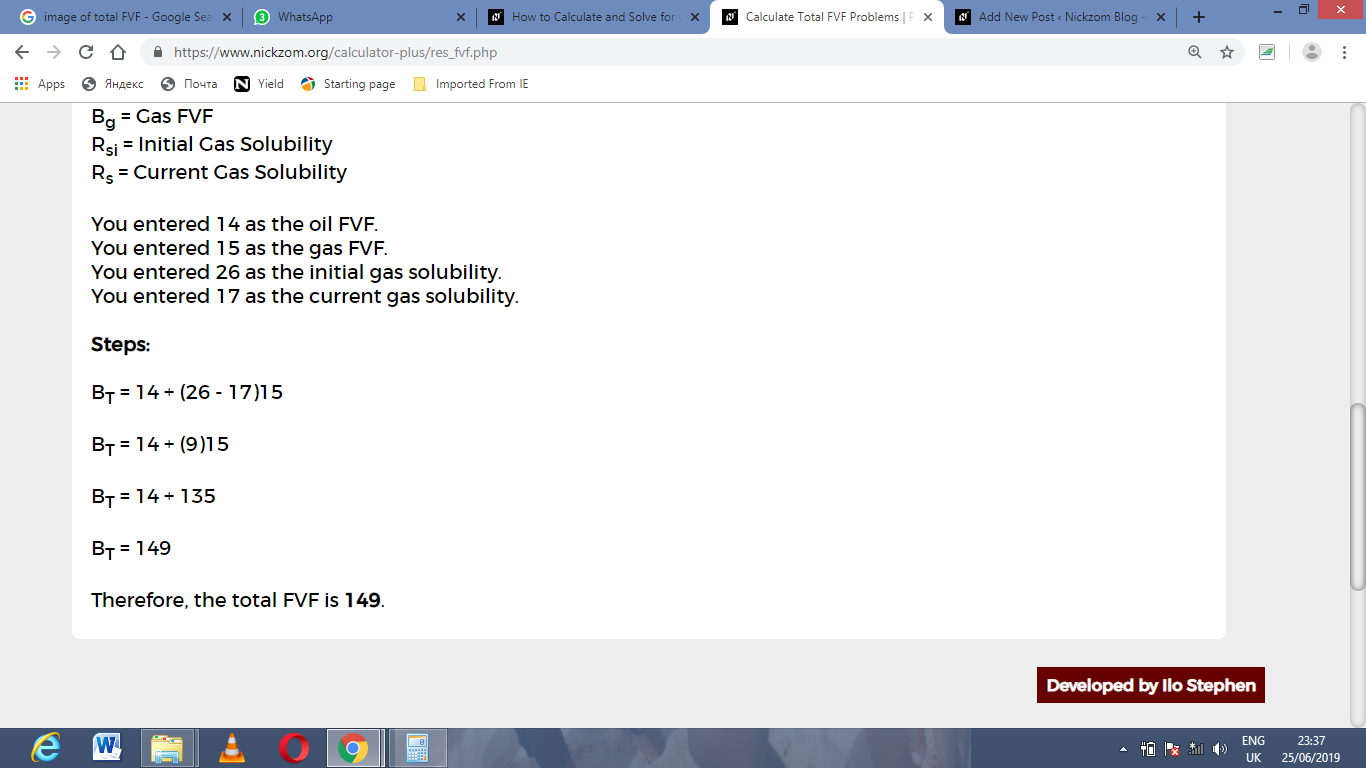As you can see from the screenshot above, Nickzom Calculator– The Calculator Encyclopedia solves for the total FVF and presents the formula, workings and steps too.